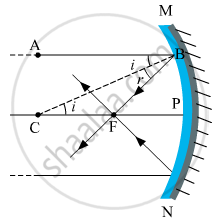# For paraxial rays, show that the focal length of a spherical mirror is one-half of its radius of curvature. - Physics

Short Note

For paraxial rays, show that the focal length of a spherical mirror is one-half of its radius of curvature.

#### SolutionConsider a ray of light AB, parallel to the principal axis and incident on a spherical mirror at point B. The normal to the surface at point B is CB and CP = CB = R is the radius of curvature. The ray AB, after reflection from the mirror, will pass through F (concave mirror) or will appear to diverge from F (convex mirror) and obeys the law of reflection i.e. i = r.
From the geometry of the figure,

In ΔCFB,∠BCP =∠ABC = i (Alternate angles)

∠CBF = r

BF = FC (because i = r)

If the aperture of the mirror is small, B lies close to P, and therefore BF = PF

Or FC = FP = PF

Or PC = PF + FC = PF + PF

Or R = 2 PF = 2f

Or "f" = "R"/2

A similar relation holds for convex mirror also. In deriving this relation, we have assumed that the aperture of the mirror is small.

Concept: Ray Optics - Mirror Formula
Is there an error in this question or solution?